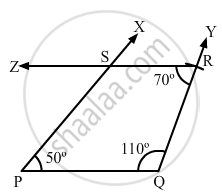Share

# Construct a Quadrilateral Pqrs, in Which Pq = 4 Cm, Qr = 5 Cm, ∠P = 50°, ∠Q = 110° and ∠R = 70°. - Mathematics

Course
ConceptConstructing a Quadrilateral When Two Adjacent Sides and Three Angles Are Known

#### Question

Construct a quadrilateral PQRS, in which PQ = 4 cm, QR = 5 cm, ∠P = 50°, ∠Q = 110° and ∠R = 70°.

#### Solution

$\text { Steps of construction: }$

$\text { Step I: Draw PQ = 4 cm }.$

$\text { Step II: Construct } \angle XPQ = 50^\circ \text { at P and } \angle PQY = 110^\circ \text { at Q }.$

$\text { Step III : With Q as the centre and radius 5 cm, cut off QR = 5 cm } .$

$\text { Step IV: At R, draw } \angle QRZ = 70^\circ \text { such that it meets PX at S } .$